﻿ 船用液压缸缓冲特性研究
 舰船科学技术2019, Vol. 41Issue (8): 114-117PDF

1. 武汉第二船舶设计研究所，湖北 武汉 430205;
2. 中国船舶重工集团公司第七二二研究所，湖北 武汉 430205

Research on cushion characteristics of marine hydraulic cylinder
LAI Qi-wei1, DUAN Wei2, XIANG Chao1, WU Chao1, WANG Qi1
1. Wuhan Second Ship Design and Research Institute, Wuhan 430205, China;
2. The 722 Research Istitute of CSIC, Wuhan 430205, China
Abstract: To solve the hydraulic shock affecting the reliability of marine hydraulic component and system during the hydraulic cylinder working process, the cushion characteristics of hydraulic cylinder served as the research object. Based on the mathematical model of hydraulic cylinder cushion process, the best structure of hydraulic cylinder has been analyzed. The dynamic simulation model of the complete hydraulic system containing hydraulic cylinder controlled by valves has been built in AMESim. The simulation results which are compared with theoretical analysis results indicate the cushion characteristics of hydraulic cylinder under different structure and initial gap. The research will be useful for understanding, utilizing and designing marine hydraulic cylinder.
Key words: hydraulic cylinder     cushion     simulation analysis     dynamic characteristics
0 引　言

1 液压缸缓冲原理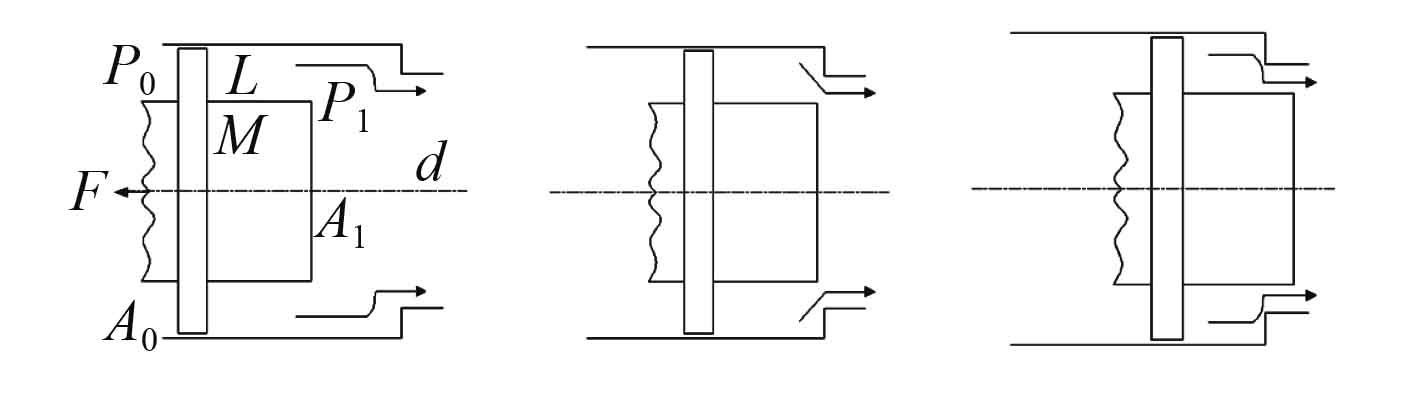图 1 液压缸缓冲过程示意图 Fig. 1 Cushion process of hydraulic cylinder diagram

 $\left\{ \begin{array}{l} M\dfrac{{{\rm{d}}V}}{{{\rm{d}}t}} = {P_0}{A_0} - {P_1}{A_1} - F{\text{，}} \\ Q = {A_1}V = {C_d}d{\text{π}} \delta \sqrt {\frac{{2{P_1}}}{\rho }} {\text{。}} \\ \end{array} \right.$ (1)

 $\left\{ \begin{array}{l} \dfrac{{{\rm{d}}V}}{{{\rm{d}}t}} + {K_1}V - {K_2} = 0 {\text{，}}\\ {K_1} = \dfrac{{\rho A_1^3}}{{2M{{\left( {{C_d}d\text{π} \delta } \right)}^2}}} {\text{，}} \\ {K_2} = \dfrac{{{P_0}{A_0} - F}}{M} {\text{。}} \end{array} \right.$ (2)

 $V = \sqrt {\frac{{{K_2}}}{{{K_1}}}} \frac{{\left( {{V_0} + \sqrt {\dfrac{{{K_2}}}{{{K_1}}}} } \right){e^{2\sqrt {{K_1}{K_2}} t}} + \left( {{V_0} - \sqrt {\dfrac{{{K_2}}}{{{K_1}}}} } \right)}}{{\left( {{V_0} + \sqrt {\dfrac{{{K_2}}}{{{K_1}}}} } \right){e^{2\sqrt {{K_1}{K_2}} t}} - \left( {{V_0} - \sqrt {\dfrac{{{K_2}}}{{{K_1}}}} } \right)}}{\text{，}}$ (3)

 ${P_1} = \frac{{\rho {A_1}^2}}{{2{{\left( {{C_d}d{\text{π}} \delta } \right)}^2}}}{V^2} {\text{，}}$ (4)

 $a = - \frac{{4{K_2}{e^{2\sqrt {{K_1}{K_2}} t}}\left( {{V_0}^2 - \dfrac{J}{K}} \right)}}{{{{\left[ {\left( {{V_0} + \sqrt {\dfrac{{{K_2}}}{{{K_1}}}} } \right){e^{2\sqrt {{K_1}{K_2}} t}} - \left( {{V_0} - \sqrt {\dfrac{{{K_2}}}{{{K_1}}}} } \right)} \right]}^2}}}{\text{，}}$ (5)

 $\left\{ \begin{array}{l} {a_{\max }} = - \left( {{K_1}{V_0}^2 - {K_2}} \right) {\text{，}} \\ {P_{\max }} = \dfrac{{\rho {A_1}^2}}{{2{{\left( {{C_d}d{\text{π}} \delta } \right)}^2}}}{V_0}^2 {\text{，}} \end{array} \right.$ (6)

 $\left\{ \begin{array}{l} V = \sqrt {{V_0}^2 - 2ax} \;{\text{，}} \\ a = \dfrac{{{P_1}{A_1} - \left( {{P_0}{A_0} - F} \right)}}{M} {\text{，}} \end{array} \right.$ (7)

 ${\delta _{(x)}}^2 = \frac{{\rho {A_1}^2\left( {{V_0}^2 - 2ax} \right)}}{{2{{\left( {{C_d}d{\text{π}} } \right)}^2}{P_1}}}{\text{。}}$ (8)

2 液压缸缓冲综合动力学仿真模型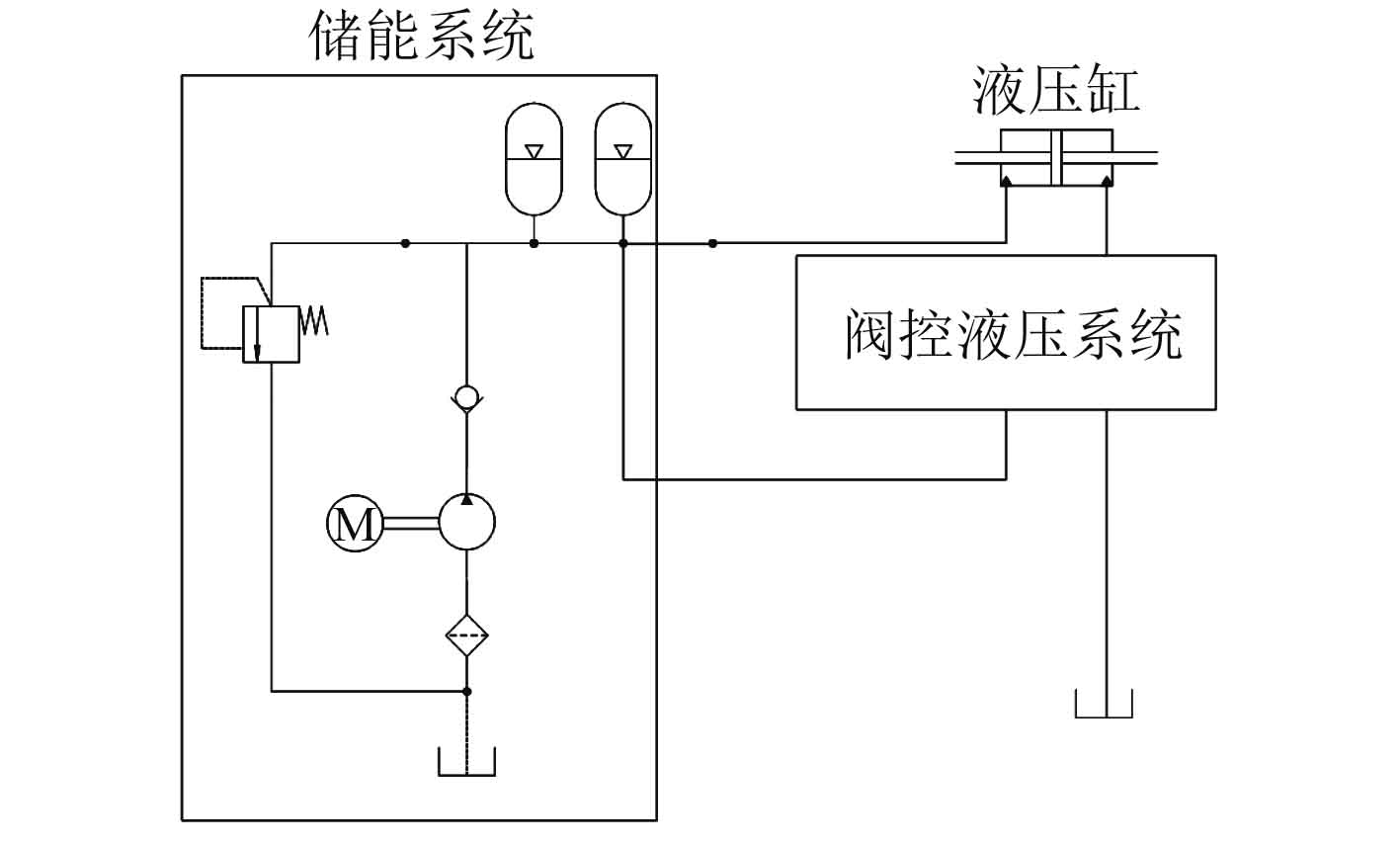图 2 阀控液压缸系统原理图 Fig. 2 Principle diagram of valve controlling cylinder hydraulic system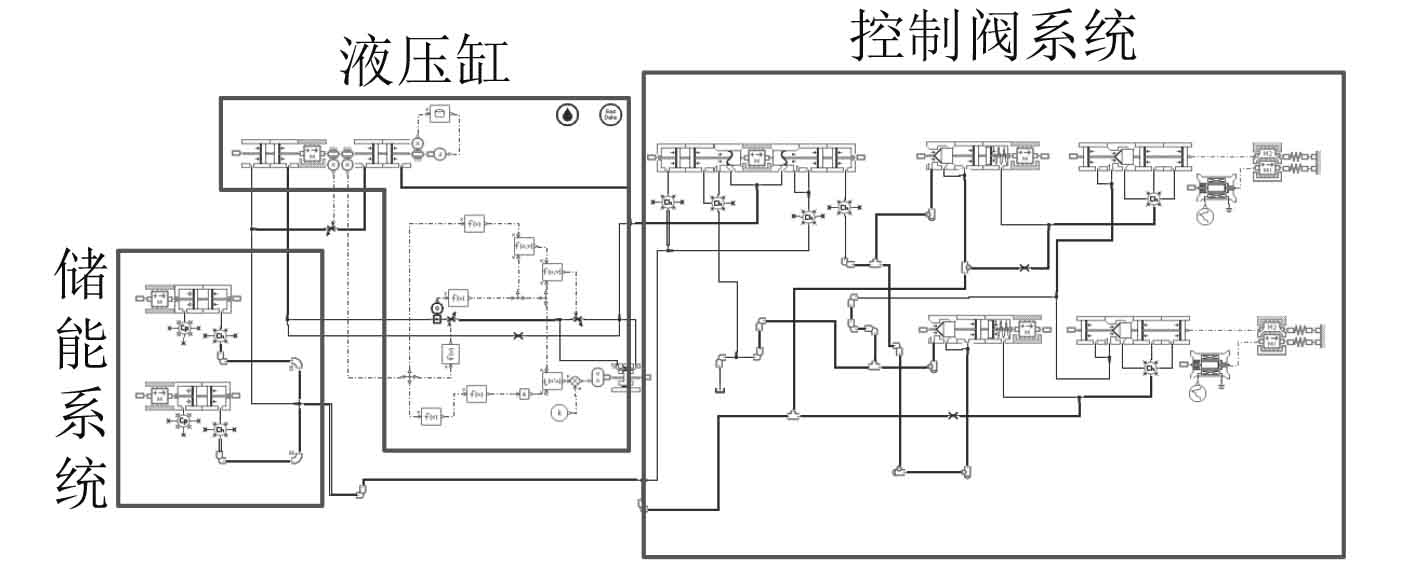图 3 阀控液压缸系统动力学仿真模型 Fig. 3 Simulation dynamic model of valve controlling cylinder hydraulic system表 1 计算所需主要参数 Tab.1 Main parameter of calculation
3 仿真分析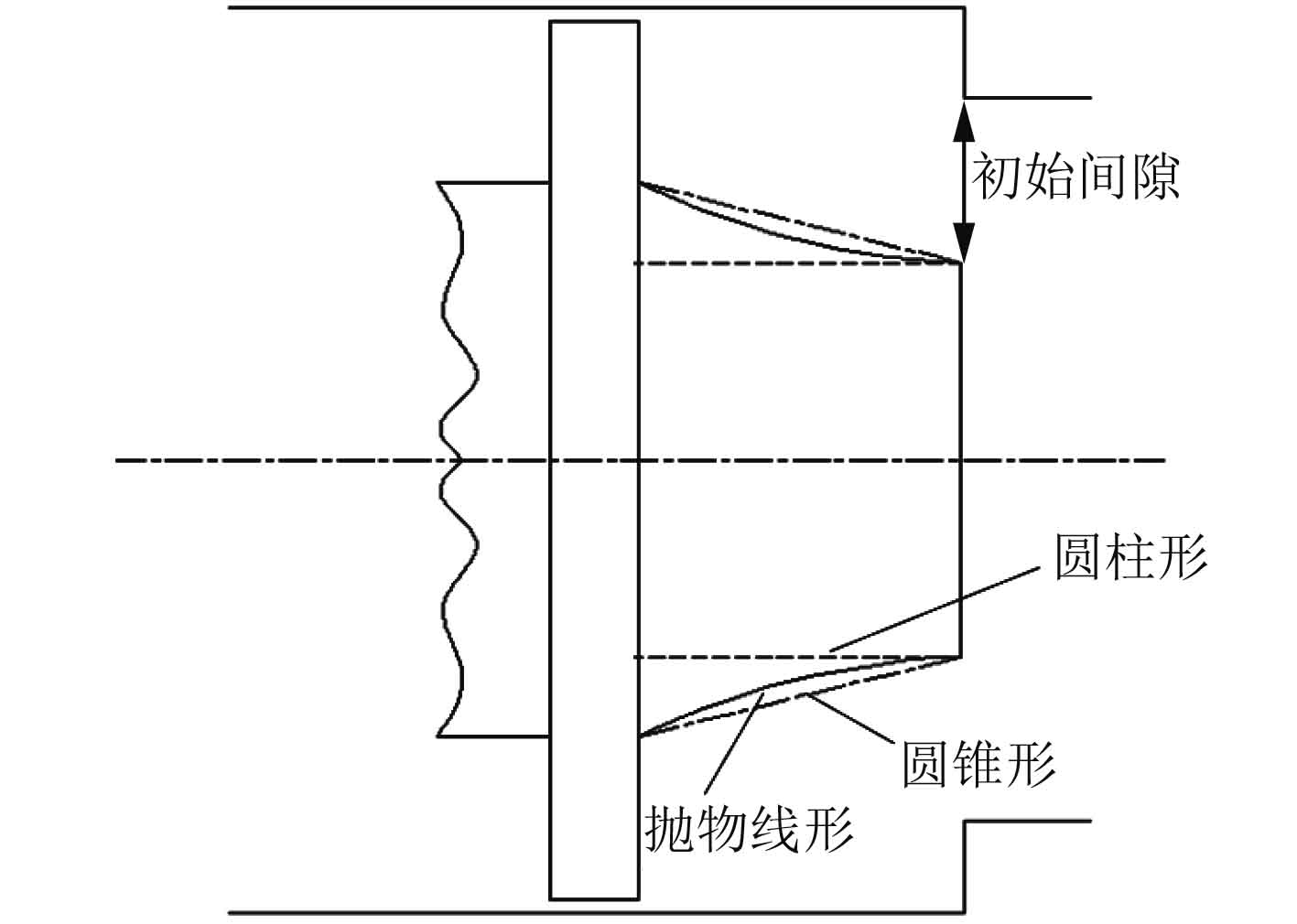图 4 不同结构形式液压缸活塞 Fig. 4 Different structure of hydraulic cylinder piston

3种活塞杆在不同初始间隙时的计算结果如图5图7表2所示。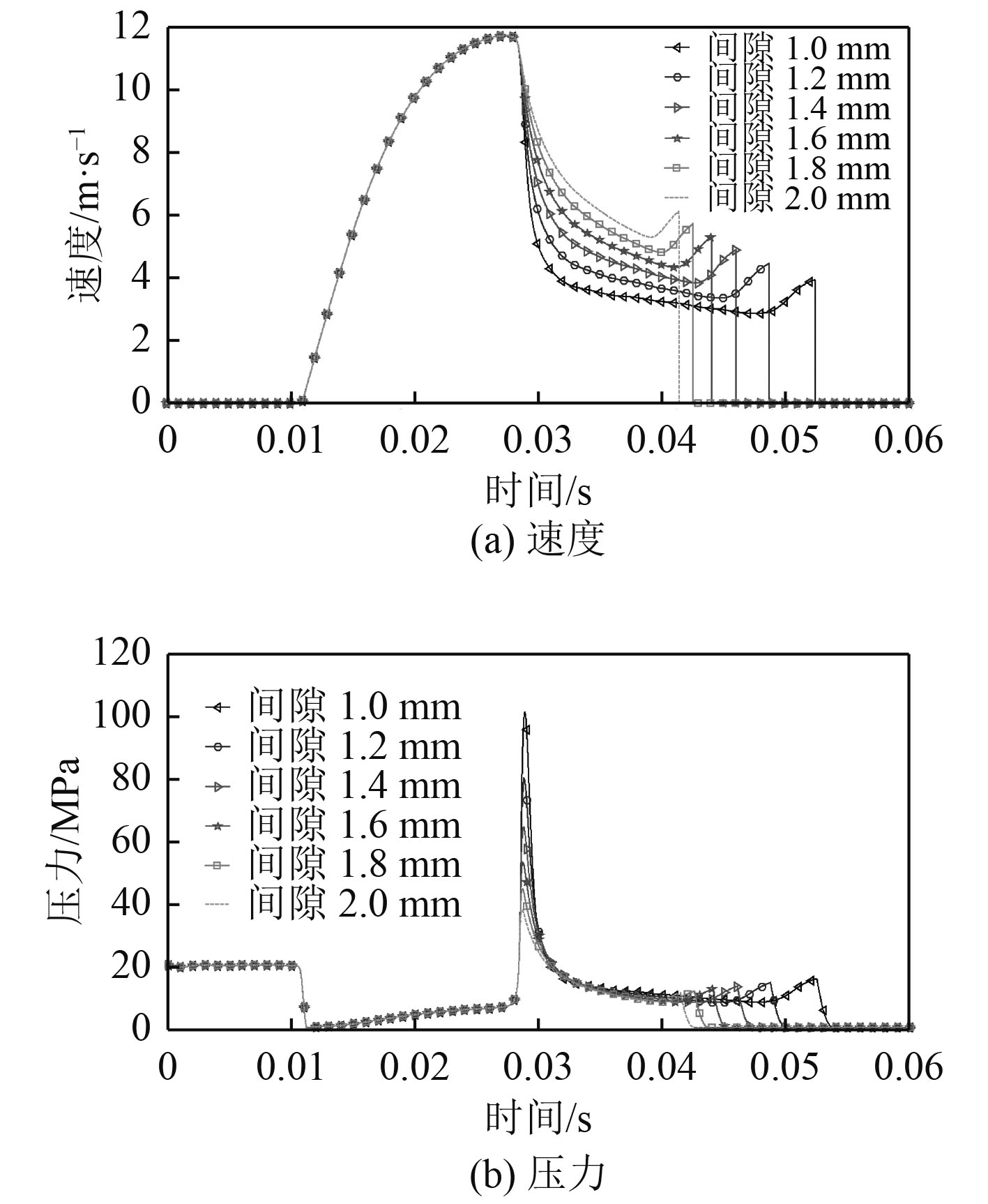图 5 圆柱形活塞杆液压缸缓冲效果 Fig. 5 Cushion effect of cylindrical piston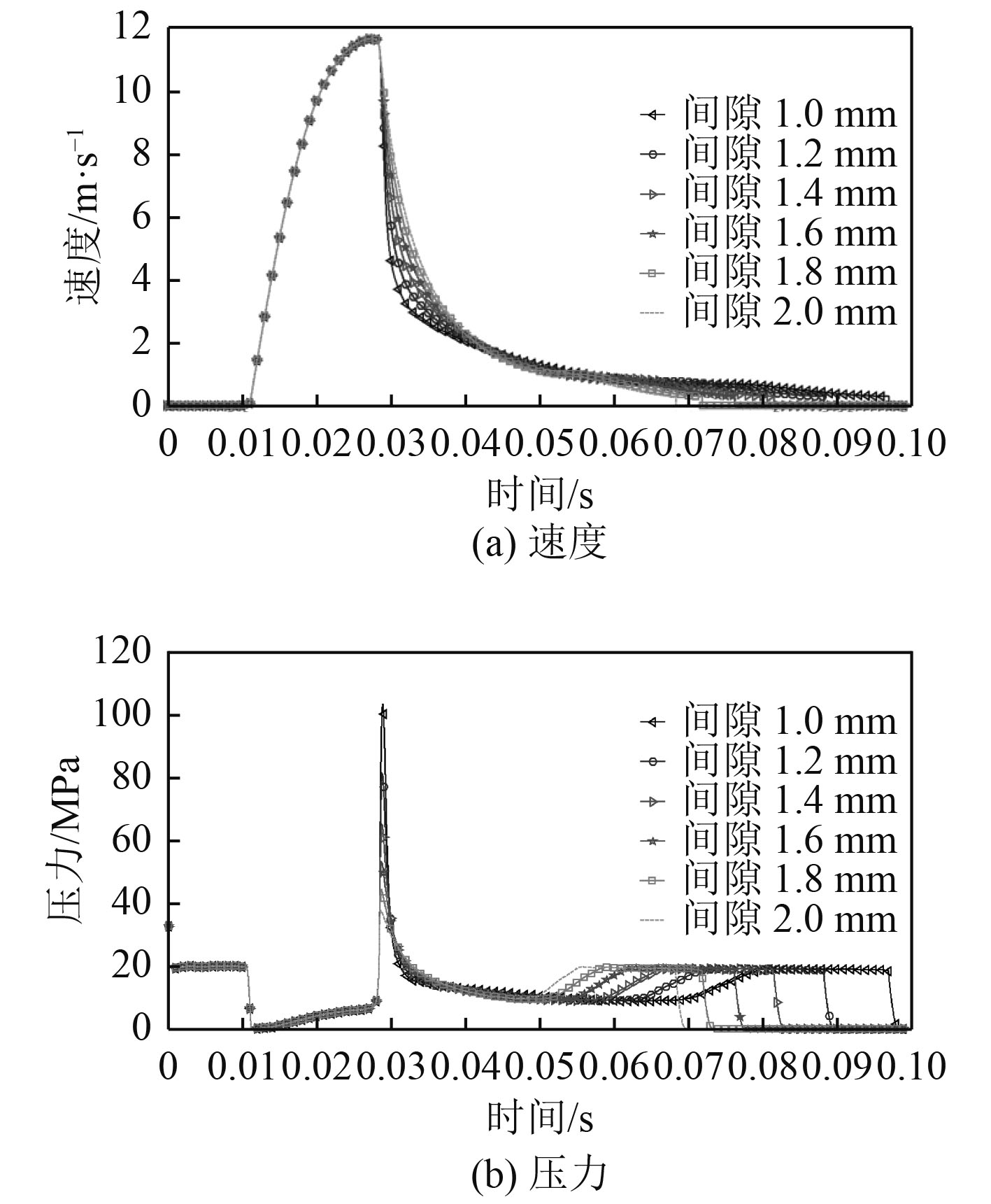图 6 圆锥形活塞杆液压缸缓冲效果 Fig. 6 Cushion effect of conical piston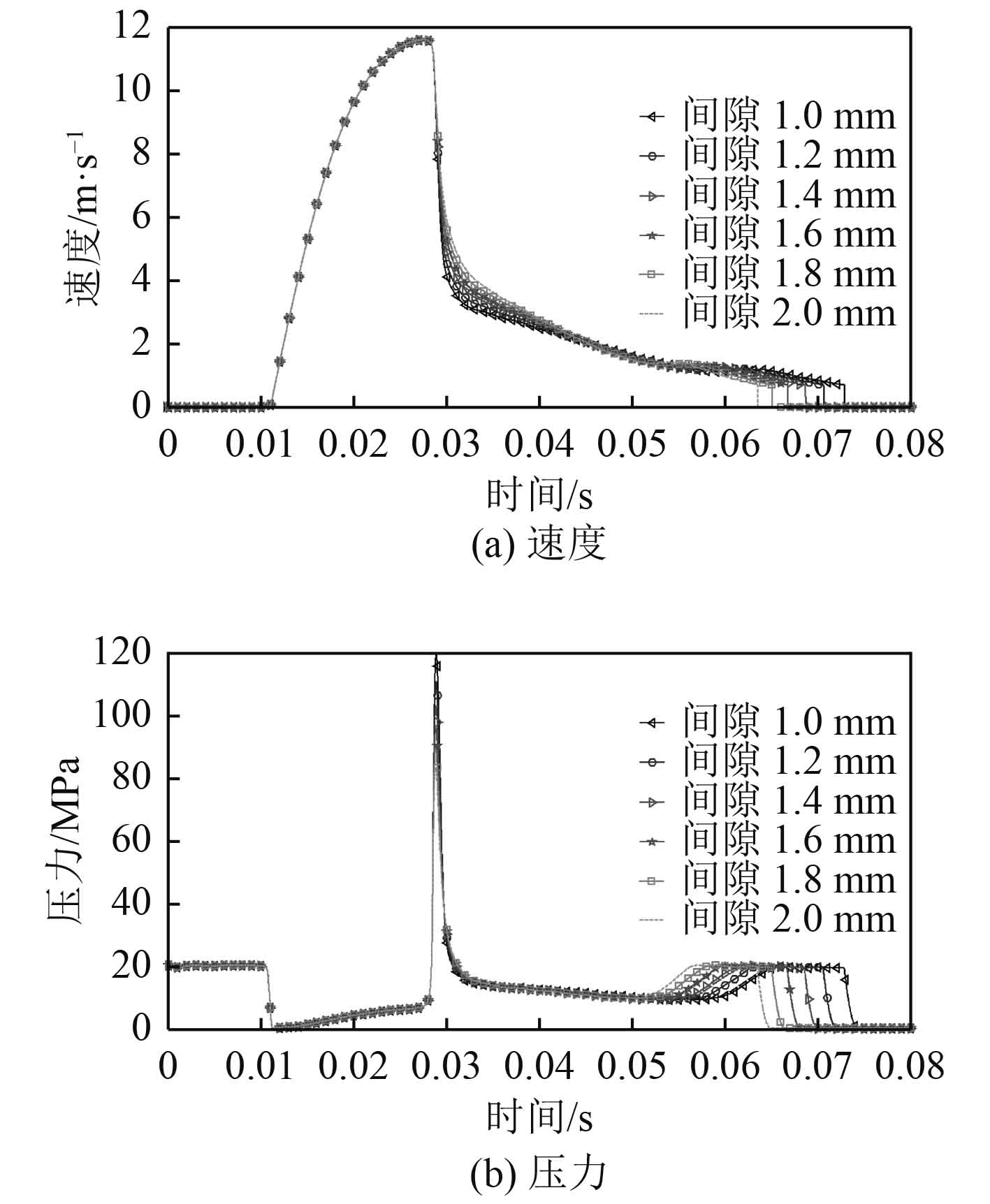图 7 抛物线形活塞杆液压缸缓冲效果 Fig. 7 Cushion effect of parabolic piston表 2 不同结构形式活塞杆液压缸缓冲效果 Tab.2 Cushion effect of different piston structure

1）对于圆柱形活塞杆，随着间隙增大，其缓冲末速度增大，且缓冲峰值压力减小。

2）对于圆锥形活塞杆，随着间隙增大，其缓冲末速度变化不明显，且缓冲峰值压力减小。相较于圆柱形活塞，圆锥形活塞杆末速度较低，但峰值压力略高。

3）对于抛物线形活塞杆，随着间隙增大，其缓冲末速度变化不明显，且缓冲峰值压力减小。其缓冲末速度普遍高于圆锥形活塞杆，但小于圆柱形活塞杆。其峰值压力普遍高于圆锥形和圆柱形活塞杆。

 $\left\{ \begin{array}{l} {E_k} + {E_p} + {E_w} = {E_{const}}{\text{，}} \\ {E_k} = \dfrac{1}{2}{M_1}V{(t)^2} {\text{，}} \\ {E_p} = \dfrac{{{M_2}P(t)}}{\rho } {\text{。}}\\ \end{array} \right.$ (9)

4 结　语

1）初始间隙值越大，不同结构形式的活塞杆均表现出缓冲峰值压力越小。间隙值对圆锥形、抛物线形缓冲末速度影响不大。

2）考虑初始间隙变化的条件下，圆锥形活塞杆具有最优的缓冲效果。

3）在工程实际中，可结合初始间隙值的计算结果，综合考虑活塞杆长度、缓冲孔直径、活塞直径来设计活塞杆结构形式，以达到最优缓冲效果。

  王洪波, 王邦勤, 王旭东. 船用活塞式液压缸故障分析[J]. 中小企业管理与科技, 2010(2): 207-208.  SOCHACKI W. Modelling and analysis of damped vibration in hydraulic cylinder[J]. Mathematical & Computer Modelling of Dynamical Systems, 2015, 21(1): 23-37.  陈宗斌, 何琳, 徐荣武. 船用操舵系统低噪声设计及特性分析[J]. 舰船科学技术, 2016, 38(7): 49-53. CHEN Zong-bin, HE Lin, XU Rong-wu. Low noise design and characteristics analysis for marine steering system[J]. Ship science and Technology, 2016, 38(7): 49-53. DOI:10.3404/j.issn.1672-7619.2016.07.011  TENG C K, HSIAO C Y, WANG C S. The effect of the guiding directions of the guiding cylinders of an area ratio modified machine cushion on the capability of suppressing impact and vibration[J]. Advances in Engineering Software, 2009, 40(10): 991-999. DOI:10.1016/j.advengsoft.2009.03.014  GHADIMI, PARVIZDASHTIMANESH, ABBASDJEDDI, et al. Study of water entry of circular cylinder by using analytical and numerical solutions[J]. Journal of the Brazilian Society of Mechanical Sciences & Engineering, 2012, 34(3): 225-232.  王成斌, 权龙. 大惯量负载液压冲击的主动变阻尼抑制方法[J]. 机械工程学报, 2014, 50(8): 182-188.  陈勋, 李丽红, 周军, 等. 高压斗杆缸缓冲性能影响参数的分析与优化研究[J]. 机电工程, 2014, 31(7): 833-838.  方秀荣, 范海峰. 液压缓冲制动缸的设计研究[J]. 机床与液压, 2010, 38(10): 43-45. FANG Xiu-rong, FAN Hai-feng. Design of the cushion and brake hydraulic cylinder[J]. Machine Tool & Hydraulics, 2010, 38(10): 43-45. DOI:10.3969/j.issn.1001-3881.2010.10.015